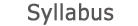2015 - 2016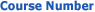0512-4200-05
Introduction to Digital Signal Processing
FACULTY OF ENGINEERING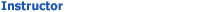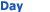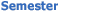Itzhak Nanikashvily Wolfson - Engineering 130 0800-1000 Sem  2 Wolfson - Engineering 134 0800-1000 Sem  2
University credit hours:  1.0

Course description
Credit Points: 3.5
Prerequisites: Introduction to Signal Analysis

• The Z transform, review and extensions. Pole zero and region of convergence (ROC) analysis, relation to stability and causality. Inverse Z transform. Transform analysis of linear time invariant (LTI) systems. Minimum phase systems.

• Discrete time processing of continuous time signals, review and extensions. Sampling rate conversions. Polyphase decompositions.
• Design of digital filters. Design of infinite impulse response (IIR) filters from analog filters. Design of finite impulse response (FIR) filters, windows and frequency sampling. Linear phase filters. Optimal (minimax) design of FIR filters.
• Discrete Fourier series (DFS). Discrete Fourier transform (DFT). Circular convolution and linear convolution using the DFT.
• The fast Fourier transform (FFT) for fast calculation of the DFT. Decimation in time and decimation in frequency FFT. The Goertzel algorithm. The chirp transform algorithm.
• The discrete cosine transform (DCT).
• Spectral analysis using short time Fourier transform.

accessibility declaration